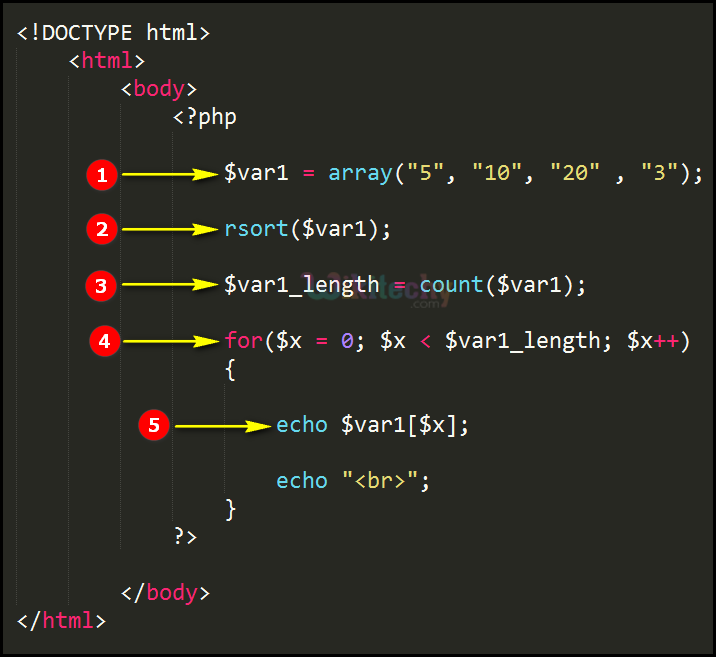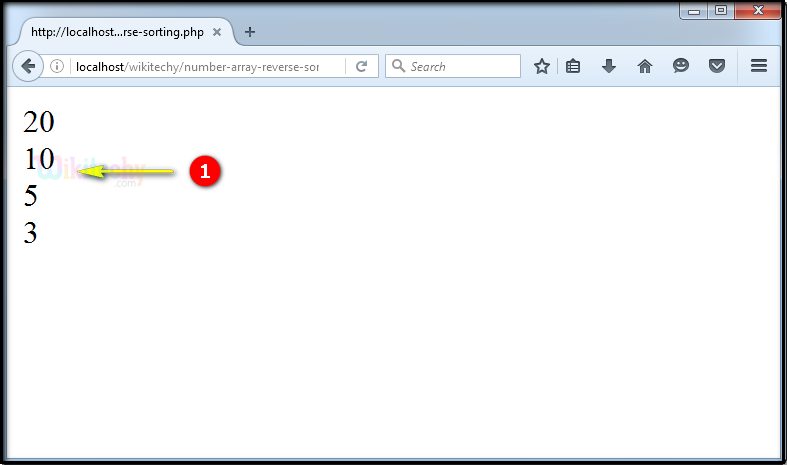# php tutorial - PHP rsort Numeric - php programming - learn php - php code - php script

• In php the rsort function is used for sorting the array in terms of descending order.
• Basically the rsort function sort the numbers in descending order.

## php program Code Explanation :1. In this statement “\$var1” is an array variable having array values “5,10,20 and 3”.
2. sort(\$var1); is the Sort function called over here which is sorting the array values (5,10,20 and 3) in descending order.
3. In this statement the count function is used for counting the number of values in the array variable “var1” which is being stored in variable \$var1_length .
4. Statement defines the for loop statement for processing the array values.
5. echo \$var1[\$x]; This echo statement will print the sorted array values from the variable “\$var1” .

## php coding Sample Output :1. Output shows the array values (5,10,20 and 3) are sorted in descending order (20,10,5 and 3) as shown here.